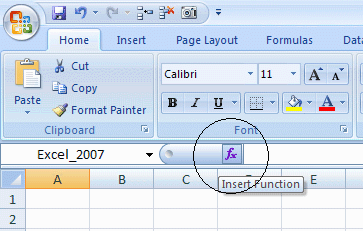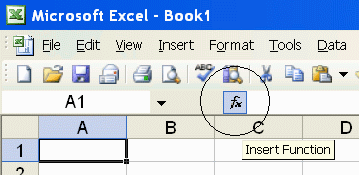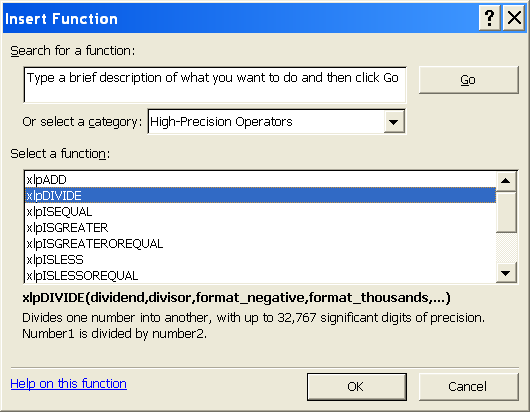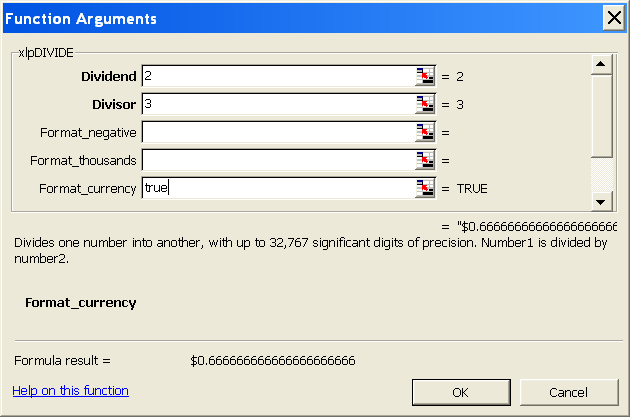PrecisionCalc
xl
Precision
Get Your Numbers Right

xlpSUBTRACT

Subtracts one number from another, with up to 32,767 significant digits of precision.

Syntax

xlpSUBTRACT(number1,number2,format_negative,format_thousands,format_currency,exponential_notation,
maximum_significant_digits,format_decimal_place)

 number1 Required. The number from which number2 will be subtracted. number2 Required. The number that will be subtracted from number1. format_negative Optional. Determines whether negatives are formatted with a leading hyphen ("-"), or parentheses ("()"). Set to 1 to format negatives with a leading hyphen. Set to 2 for parentheses. 1 by default. You can also format negatives with a red font. format_thousands Optional. Determines whether thousands separators are included. Set to TRUE to include thousands separators. FALSE by default. format_currency Optional. Determines whether currency symbol is included. Set to TRUE to include currency symbol. The currency symbol will be added either to the beginning or to the end of the result, whichever is appropriate for the locale. FALSE by default. exponential_notation Optional. Determines whether result is formatted in exponential notation. Set to TRUE to format in exponential notation. FALSE by default. maximum_significant_digits Optional. Determines the maximum number of significant digits to be returned. Default is 100, or the user's custom maximum set in the About box, or the maximum number allowed by the edition of xlPrecision, whichever is less. format_decimal_place Optional. Determines whether to add or remove decimal places as required to return the exact specified number of decimal places.When format_decimal_place causes decimal places to be removed, the remaining decimals are rounded, exactly as Excel's number formatting does. Set format_decimal_place to the number of decimal places desired. For example, set to 3 to specify that the result should have exactly 3 decimal places, so that: "123.4" is formatted as "123.400" "12.3456" is formatted as "12.346" "9.9999" is formatted as "10.000"

Use the Insert Function Dialog to easily enter these arguments:
(available on most editions of xlPrecision, but not recommended with the Free Edition as it causes the Free Edition dialog to appear multiple times)Examples

 Formula Description Result =xlpSUBTRACT(1,2) 1 - 2 -1 =xlpSUBTRACT("123456789123.456789",                       "123456.789123456789") To prevent Excel from truncating the numbers to 15 digits, enter them in quotes as shown. 123456789123.456789 - 123456.789123456789, without letting Excel truncate to 15 significant digits 123456665666.667665543211 (25 significant digits) =xlpSUBTRACT(1,2,2) 1 - 2, formatted with parentheses if negative (1) =xlpSUBTRACT(2000,2,,TRUE) 2,000 - 2, formatted with localized thousands separators 1,998  (in the USA) 1.998  (in Germany) 1 998  (in France) =xlpSUBTRACT(2000,2,,,TRUE) 2,000 - 2, formatted with the local currency symbol \$1,998  (in the USA) 1.998 €  (in Germany) 1 998 €  (in France) 1.998 kr  (in Estonia) 1,998Lek  (in Albania) =xlpSUBTRACT(2000,2,,,,TRUE) 2,000 - 2, formatted in exponential notation 1.998E+3 =xlpSUBTRACT("1.002E+3","3.004E+3",,,,TRUE) '1.002E+3' - '3.004E+3', formatted in exponential notation -2.002E+3 =xlpSUBTRACT(A1,A2,,,,,500) =xlpSUBTRACT(A1,A2,,,,,500000) =xlpSUBTRACT(A1,A2,,,,,A3) To prevent Excel from truncating the numbers in cells A1 & A2 to 15 digits, preformat those cells as "Text" number format, or enter a leading apostrophe. A1 - A2, with up to 500 (or 500,000, or the number in A3) significant digits of precision. Allows A1, A2, and the return value to each be up to 500 (or 500,000, or the number in A3) significant digits. =xlpSUBTRACT(12.3,23,,,,,,3) 12.3 - 23, formatted to 3 decimal places. -10.700 (padded with zeros to meet the specified number of decimal places) =xlpSUBTRACT(12.3,0.123,,,,,,2) 12.3 - 0.123, formatted to 2 decimal places. 12.18 (rounded to fit the specified number of decimal places) =xlpSUBTRACT(2,2000,2,TRUE,TRUE,,500,2) 2 - 2,000, formatted with: parentheses if negative localized thousands separators local currency symbol up to 500 significant digits of precision 2 decimal places (\$1,998.00)  (in the USA) (1.998,00 €)  (in Germany) (1 998,00 €)  (in France) (1 998.00 kr)  (in Estonia) (1.998,00Lek)  (in Albania)

Remarks

• xlPrecision results are returned as text that look like numbers, not as values that Excel recognizes as numbers. This is because Excel would truncate the results to 15 significant digits if it recognized them as numbers.

• Some arguments are ignored in some editions of xlPrecison. See the Buy page for details.

• maximum_significant_digits is ignored if it is higher than the maximum significant digits allowed by the edition of xlPrecision.

• Use maximum_significant_digits to increase calculation speed where desired. The lower the number used, the faster the calculation.

• number1 and number2 can accept both numbers and text.

• number1 and number2 can accept text formatted with the local currency symbol and thousands separators, and negatives can be formatted with either a leading hyphen or parentheses. They also can accept numbers formatted in scientific notation.

• number1 and number2 can accept text up to 32,767 characters long, depending on the edition of xlPrecision.

• You can use the results of xlPrecision functions as the operands in other xlPrecision formulas without losing any precision, but using them as operands in Excel's arithmetic functions will truncate them to 15 significant digits.

• If the return value is so large that it has more than 32,767 characters to the left of the decimal, then xlPrecision is of course unable to return a correct value and instead returns "#VALUE!". To return that data successfully, set exponential_notation to TRUE to return the value in exponential notation.

• 32,767 digits to the left of the decimal is a vastly larger number than Excel can return without xlPrecision. Excel itself can only return or recognize a number with no more than 308 digits to the left of the decimal.

• Decimal symbols, thousands separators, and currency symbols are all localized. This means that an xlPrecision formula that returns "\$1,234,567.89" in the USA will return "1.234.567,89 €" in Germany, "1 234 567,89 €" in France, "1 234 567.89 kr" in Estonia, and "1.234.567,89Lek" in Albania.

• The 32,767 SD edition can only provide a maximum of 32,767 total characters, including all formatting characters such as decimal, leading hyphen or parentheses for negatives, and thousands separators. As a result, it can only return the maximum 32,767 significant digits when the result is an unformatted positive integer. This is due to Excel's limitation of 32,767 characters in a cell. In all cases, the 32,767 SD edition will give you as many significant digits as possible with the formatting you have chosen.

• Depending on how many significant digits the edition of xlPrecision provides, the result may be too long to conveniently view. You can view the full result by right-clicking the cell and choosing Format Cells | Alignment | Wrap Text, and widening the column to the width of the screen. An easy way to view the full result without changing column widths or wrapping text is to right-click the cell, choose Copy, and then paste into Notepad or a word processor.